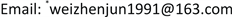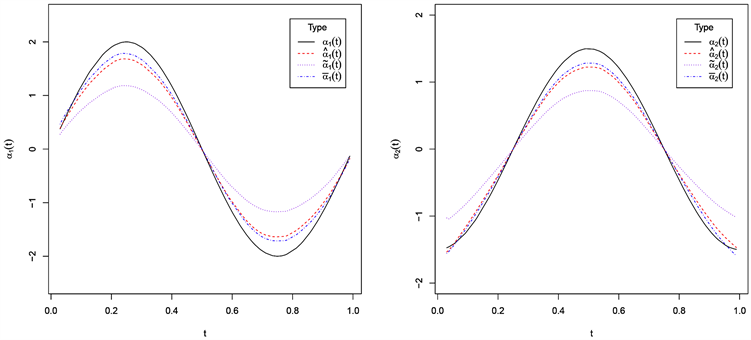﻿ 缺失数据下部分线性变系数EV模型在生鲜产品销售量预测中的应用 Application of Partial Linear Variable Coefficient EV Model in Fresh Product Sales Forecast under Missing Data

Statistics and Application
Vol. 09  No. 01 ( 2020 ), Article ID: 34064 , 10 pages
10.12677/SA.2020.91007

Application of Partial Linear Variable Coefficient EV Model in Fresh Product Sales Forecast under Missing Data

Zhenjun Wei*, Yanhui Gong, Chunliu Li

Trial Retail Engineering Co. Ltd., Yantai ShandongReceived: Dec. 30th, 2019; accepted: Jan. 14th, 2020; published: Jan. 21st, 2020ABSTRACT

In this paper, we mainly consider the statistical inference for partially linear varying coefficient errors in variables models in the nonparametric part and the responses are missing at random. Based on local linear smoothing techniques, profile least-squares and bias-corrected methods, we obtained estimators successfully about both parametric and nonparametric components. Besides, to avoid to estimate the asymptotic covariance in establishing confidence region of the parametric component with the normal-approximation method, we define an empirical likelihood based statistic. Then, the confidence regions of the parametric component with asymptotically correct coverage probabilities can be constructed by the result. The simulation results show that the empirical likelihood method has better finite sample properties compared with the normal approximation method. Finally, the method is applied to a real data analysis of the supermarket fresh sales volume data and gives better estimation.

Keywords:Missing Data, Partially Linear Varying Coefficient Model, Variable with Measurement Errors, Empirical-Likelihood1. 引言

$Y={X}^{\tau }\beta +{Z}^{\tau }\alpha \left(T\right)+\epsilon ,$ (1.1)

$\left\{\left({X}_{i},{Y}_{i},{\delta }_{i}\right),1\le i\le n\right\},$ (1.2)

$Pr\left(\delta =1|Y,X\right)=Pr\left(\delta =1|X\right),$ (1.3)

${W}_{i}={Z}_{i}+{U}_{i},i=1,2,\cdots ,n.$ (1.4)

2. 模型与参数估计

${\delta }_{i}{Y}_{i}={\delta }_{i}{X}_{i}^{\tau }\beta +{\delta }_{i}{Z}_{i}^{\tau }\alpha \left({T}_{i}\right)+{\delta }_{i}{\epsilon }_{i},i=1,2,\cdots ,n,$ (2.1)

${\delta }_{i}\left({Y}_{i}-{X}_{i}^{\tau }\beta \right)={\delta }_{i}{\alpha }_{1}\left({T}_{i}\right){Z}_{i1}+{\delta }_{i}{\alpha }_{2}\left({T}_{i}\right){Z}_{i2}+\cdots +{\delta }_{i}{\alpha }_{p}\left({T}_{i}\right){Z}_{ip}+{\delta }_{i}{\epsilon }_{i},$ (2.2)

${\alpha }_{j}\left(T\right)\approx {\alpha }_{j}\left(t\right)+{{\alpha }^{\prime }}_{j}\left(t\right)\left(T-t\right)\equiv {a}_{j}+{b}_{j}\left(T-t\right),j=1,2,\cdots ,q,$ (2.3)

$\underset{i=1}{\overset{n}{\sum }}{\left\{\left({Y}_{i}-{X}_{i}^{\tau }\beta \right)-\underset{j=1}{\overset{p}{\sum }}\left[{a}_{j}+{b}_{j}\left({T}_{i}-t\right)\right]{Z}_{ij}\right\}}^{2}{K}_{h}\left({T}_{i}-t\right){\delta }_{i},$ (2.4)

${D}_{t}^{Z}=\left[\begin{array}{cc}{Z}_{1}^{\tau }& \frac{{T}_{1}-t}{h}{Z}_{1}^{\tau }\\ ⋮& ⋮\\ {Z}_{n}^{\tau }& \frac{{T}_{n}-t}{h}{Z}_{n}^{\tau }\end{array}\right],M=\left[\begin{array}{c}{Z}_{1}^{\tau }\alpha \left({T}_{1}\right)\\ ⋮\\ {Z}_{n}^{\tau }\alpha \left({T}_{n}\right)\end{array}\right],$

${\left[{\stackrel{^}{a}}^{\tau },h{\stackrel{^}{b}}^{\tau }\right]}^{\tau }={\left\{{\left({D}_{t}^{Z}\right)}^{\tau }{\omega }_{t}^{\delta }{D}_{t}^{Z}\right\}}^{-1}{\left({D}_{t}^{Z}\right)}^{\tau }{\omega }_{t}^{\delta }\left(Y-X\beta \right),$ (2.5)

${\left[{\stackrel{^}{a}}^{\tau },h{\stackrel{^}{b}}^{\tau }\right]}^{\tau }={\left\{{\left({D}_{t}^{W}\right)}^{\tau }{\omega }_{t}^{\delta }{D}_{t}^{W}-{\Omega }^{\delta }\right\}}^{-1}{\left({D}_{t}^{W}\right)}^{\tau }{\omega }_{t}^{\delta }\left(Y-X\beta \right),$ (2.6)

${\Omega }^{\delta }=\underset{i=1}{\overset{n}{\sum }}\text{ }\text{ }{\Sigma }_{u}\otimes \left[\begin{array}{cc}1& \frac{{T}_{i}-t}{h}\\ \frac{{T}_{i}-t}{h}& {\left(\frac{{T}_{i}-t}{h}\right)}^{2}\end{array}\right]{K}_{h}\left({T}_{i}-t\right){\delta }_{i},$

$\stackrel{˜}{\alpha }\left(t\right)=\left({I}_{q}{0}_{q}\right){\left\{{\left({D}_{t}^{W}\right)}^{\tau }{\omega }_{t}^{\delta }{D}_{t}^{W}-{\Omega }^{\delta }\right\}}^{-1}{\left({D}_{t}^{W}\right)}^{\tau }{\omega }_{t}^{\delta }\left(Y-X\beta \right),$ (2.7)

$\underset{i=1}{\overset{n}{\sum }}\text{ }\text{ }{\delta }_{i}{\left\{{Y}_{i}-{X}_{i}^{\tau }\beta -{W}_{i}^{\tau }\stackrel{^}{\alpha }\left({T}_{i}\right)\right\}}^{2}-\underset{i=1}{\overset{n}{\sum }}\text{ }\text{ }{\delta }_{i}{\stackrel{^}{\alpha }}^{\tau }\left({T}_{i}\right){\Sigma }_{u}\stackrel{^}{\alpha }\left({T}_{i}\right),$ (2.8)

$\stackrel{^}{\beta }={\left\{\underset{i=1}{\overset{n}{\sum }}\text{ }\text{ }{\delta }_{i}\left({\stackrel{˜}{X}}_{i}{\stackrel{˜}{X}}_{i}^{\tau }-{X}^{\tau }{Q}_{i}^{\tau }{\Sigma }_{u}{Q}_{i}X\right)\right\}}^{-1}\left\{\underset{i=1}{\overset{n}{\sum }}\text{ }\text{ }{\delta }_{i}\left({\stackrel{˜}{X}}_{i}{\stackrel{˜}{Y}}_{i}-{X}^{\tau }{Q}_{i}^{\tau }{\Sigma }_{u}{Q}_{i}Y\right)\right\},$ (2.9)

$\stackrel{^}{\beta }={\left\{{\stackrel{˜}{X}}^{\tau }\Delta \stackrel{˜}{X}-{X}^{\tau }{Q}^{\tau }\Delta \otimes {\Sigma }_{u}QX\right\}}^{-1}\left\{{\stackrel{˜}{X}}^{\tau }\Delta \stackrel{˜}{Y}-{X}^{\tau }{Q}^{\tau }\Delta \otimes {\Sigma }_{u}QY\right\},$

$\stackrel{^}{\alpha }\left(t\right)=\left({I}_{q}{0}_{q}\right){\left\{{\left({D}_{t}^{W}\right)}^{\tau }{\omega }_{t}^{\delta }{D}_{t}^{W}-{\Omega }^{\delta }\right\}}^{-1}{\left({D}_{t}^{W}\right)}^{\tau }{\omega }_{t}^{\delta }\left(Y-X\stackrel{^}{\beta }\right).$ (2.10)

3. 经验似然推断

$\underset{i=1}{\overset{n}{\sum }}\text{ }\text{ }{\delta }_{i}\left[{\stackrel{˜}{X}}_{i}\left({\stackrel{˜}{Y}}_{i}-{\stackrel{˜}{X}}_{i}^{\tau }\beta \right)-{X}^{\tau }{Q}_{i}^{\tau }{\Sigma }_{u}{Q}_{i}\left(Y-X\beta \right)\right]=0,$ (3.1)

${\eta }_{i}\left(\beta \right)={\delta }_{i}{\stackrel{˜}{X}}_{i}\left({\stackrel{˜}{Y}}_{i}-{\stackrel{˜}{X}}_{i}^{\tau }\beta \right)-{\delta }_{i}{X}^{\tau }{Q}_{i}^{\tau }{\Sigma }_{u}{Q}_{i}\left(Y-X\beta \right),$ (3.2)

$\mathcal{L}\left(\beta \right)=-2\mathrm{max}\left\{\underset{i=1}{\overset{n}{\sum }}\mathrm{log}\left(n{p}_{i}\right)|{p}_{i}\ge 0,\underset{i=1}{\overset{n}{\sum }}\text{ }\text{ }{p}_{i}=1,\underset{i=1}{\overset{n}{\sum }}\text{ }\text{ }{p}_{i}{\eta }_{i}\left(\beta \right)=0\right\},$ (3.3)

${p}_{i}=\frac{1}{n}\frac{1}{1+{\lambda }^{\tau }{\eta }_{i}\left(\beta \right)},$ (3.4)

$\frac{1}{n}\underset{i=1}{\overset{n}{\sum }}\frac{{\eta }_{i}\left(\beta \right)}{1+{\lambda }^{\tau }{\eta }_{i}\left(\beta \right)}=0.$ (3.5)

$\mathcal{L}\left(\beta \right)=2\underset{i=1}{\overset{n}{\sum }}\mathrm{log}\left\{1+{\lambda }^{\tau }{\eta }_{i}\left(\beta \right)\right\}.$ (3.6)

4. 模拟研究

$\left(\begin{array}{l}{y}_{i}={x}_{i}\beta +{z}_{1i}{\alpha }_{1}\left({T}_{i}\right)+{z}_{2i}{\alpha }_{2}\left({T}_{i}\right)+{\epsilon }_{i},\\ {w}_{1i}={z}_{1i}+{u}_{1i},\\ {w}_{2i}={z}_{2i}+{u}_{2i},\end{array}\text{\hspace{0.17em}}\text{\hspace{0.17em}}\text{\hspace{0.17em}}\text{\hspace{0.17em}}i=1,2,\cdots ,n,$

$\text{CV}\left(h\right)=\frac{1}{n}\underset{i=1}{\overset{n}{\sum }}\text{ }{\delta }_{i}{\left\{{Y}_{i}-{X}_{i}^{\tau }{\stackrel{^}{\beta }}^{\left(-i\right)}-{W}_{i}^{\tau }{\stackrel{^}{\alpha }}_{h,\left(-i\right)}\left({T}_{i}\right)\right\}}^{2}-\frac{1}{n}\underset{i=1}{\overset{n}{\sum }}\text{ }{\delta }_{i}{\stackrel{^}{\alpha }}_{h,\left(-i\right)}^{\tau }\left({T}_{i}\right){\Sigma }_{u}{\stackrel{^}{\alpha }}_{h,\left(-i\right)}\left({T}_{i}\right),$

Case I $Pr\left(\delta =1|X=x,Z=z,T=t\right)=0.8$，对所有的 $x,z,t$

Case II $Pr\left(\delta =1|X=x,Z=z,T=t\right)=0.8+0.2\left(|x|+|t-0.5|\right)$，当 $|x|+|t-0.5|\le 1$ 时，否则取0.88。

1) 不含缺失数据：

$\stackrel{¯}{\beta }={\left\{{\stackrel{˜}{X}}^{\tau }\stackrel{˜}{X}-{X}^{\tau }{Q}^{\tau }I\otimes {\Sigma }_{u}QX\right\}}^{-1}\left\{{\stackrel{˜}{X}}^{\tau }\stackrel{˜}{Y}-{X}^{\tau }{Q}^{\tau }I\otimes {\Sigma }_{u}QY\right\},$

$\stackrel{¯}{\alpha }\left(t\right)=\left({I}_{q}{0}_{q}\right){\left\{{\left({D}_{t}^{W}\right)}^{\tau }{\omega }_{t}{D}_{t}^{W}-\Omega \right\}}^{-1}{\left({D}_{t}^{W}\right)}^{\tau }{\omega }_{t}\left(Y-X\stackrel{¯}{\beta }\right),$

2) 非参数分量含测量误差不纠偏：

$\stackrel{˜}{\beta }={\left[\underset{i=1}{\overset{n}{\sum }}\text{ }\text{ }{\delta }_{i}{\stackrel{˜}{X}}_{i}{\stackrel{˜}{X}}_{i}^{\tau }\right]}^{-1}\left[\underset{i=1}{\overset{n}{\sum }}\text{ }\text{ }{\delta }_{i}{\stackrel{˜}{X}}_{i}{\stackrel{˜}{Y}}_{i}\right],$

$\stackrel{˜}{\alpha }\left(t\right)=\left({I}_{q}{0}_{q}\right){\left\{{\left({D}_{t}^{W}\right)}^{\tau }{\omega }_{t}^{\delta }{D}_{t}^{W}\right\}}^{-1}{\left({D}_{t}^{W}\right)}^{\tau }{\omega }_{t}^{\delta }\left(Y-X\stackrel{˜}{\beta }\right),$Table 1. Mean, SD and MSE of β ^ under different conditionsTable 2. Mean, SD and MSE of β ¯ under different conditionsTable 3. Mean, SD and MSE of β ˜ under different conditionsTable 4. Average length and coverage probability of β confidence interval for 95% confidence level under different conditions

1) 在缺失概率和测量误差协方差给定的情况下，随着样本的增加，参数估计量的标准差和均方误差都逐渐减小；

2) 在缺失概率和样本量给定的情况下，测量误差协方差越小，参数估计量的标准差和均方误差越小；

3) 在测量误差协方差和样本量给定的情况下，缺失概率越小，参数估计量的标准差和均方误差越小。

1) 在对含测量误差不做任何处理时，得到的结果偏差较大，不能使人满意。

2) 本文所提的方法对含有测量误差数据的处理有很好的效果。

1) EL (经验似然法)比NA (正态近似法)有更短的置信区间和更高的覆盖率；

2) 对给定的缺失概率，随着样本量的增加，经验似然和正态近似的置信区间均会缩短；

3) 对给定的样本量，随着缺失概率的增加，经验似然和正态近似的置信区间均会增长；

4) 对给定的样本量和缺失概率，误差方差越大，不论是经验似然还是正态近似的置信区间均会增长，且覆盖率会下降。Figure 1. Real curve and all kinds of estimation curves of coefficient function (The left picture is ${\alpha }_{1}\left(t\right)$, right picture is ${\alpha }_{2}\left(t\right)\right)$

5. 实例分析

${Z}_{1}=1$ 为截距项，协变量 $T=\sqrt{\text{SWC}}$。研究讨论了 ${Z}_{2},{Z}_{3},{Z}_{4},{Z}_{5},{Z}_{6},{Z}_{7},{X}_{1},{X}_{2}$ 以及SWC对超市生鲜产品销售量的影响，并采用部分线性变系数模型

$Y=\underset{i=1}{\overset{7}{\sum }}\text{ }\text{ }{\alpha }_{i}\left(T\right){Z}_{i}+{\beta }_{1}{X}_{1}+{\beta }_{2}{X}_{2}+\epsilon$

${W}_{6}={Z}_{6}+{U}_{6}$

Application of Partial Linear Variable Coefficient EV Model in Fresh Product Sales Forecast under Missing Data[J]. 统计学与应用, 2020, 09(01): 53-62. https://doi.org/10.12677/SA.2020.91007

1. 1. Engle, R.F., Granger, C.W.J., Rice, J.J. and Weiss, A. (1986) Semiparametric Estimates of the Relation between Weather and Electricity Sales. Journal of the American Statistical Association, 81, 310-320. https://doi.org/10.1080/01621459.1986.10478274

2. 2. Fan, J.Q. and Huang, T. (2005) Profile Likelihood Inferences on Semiparametric Varying-Coefficient Partially Linear Models. Bernoulli, 11, 1031-1057. https://doi.org/10.3150/bj/1137421639

3. 3. Huang, Z.S. and Zhang, R.Q. (2009) Empirical Likelihood for Nonparametric Parts in Semiparametric Varying-Coefficient Partially Linear Models. Statistics and Probability Letters, 79, 1798-1808. https://doi.org/10.1016/j.spl.2009.05.008

4. 4. Wei, C.H. (2012) Statistical Inference in Partially Linear Varying-Coefficient Models with Missing Responses at Random. Communications in Statistics-Theory and Methods, 41, 1284-1298. https://doi.org/10.1080/03610926.2010.542854

5. 5. Wei, C.H. and Mei, C.L. (2012) Empirical Likelihood for Partially Linear Varying-Coefficient Models with Missing Response Variables and Error-Prone Covariates. Journal of the Korean Statistical Society, 41, 97-103. https://doi.org/10.1016/j.jkss.2011.06.004

6. 6. Yang, Y.P., Xue, L.G. and Cheng, W.H. (2011) Two-Step Estimators in Partial Linear Models with Missing Response Variables and Error-Prone Covariates. Journal of Systems Science and Complexity, 24, 1165-1182. https://doi.org/10.1007/s11424-011-8393-9

7. 7. You, J.H. and Chen, G.M. (2006) Estimation of a Semiparametric Varying-Coefficient Partially Linear Errors-in-Variables Model. Journal of Multivariate Analysis, 97, 324-341. https://doi.org/10.1016/j.jmva.2005.03.002

8. 8. Zhang, W.W., Li, G.R. and Xue, L.G. (2011) Profile Inference on Partially Linear Varying-Coefficient Errors-in-Variables Models under Restricted Condition. Computational Statistics and Data Analysis, 55, 3027-3040. https://doi.org/10.1016/j.csda.2011.05.012

9. 9. Fan, G.L., Liang, H.Y. and Shen, Y. (2016) Penalized Empirical Likelihood for High-Dimensional Partially Linear Varying Coefficient Model with Measurement Errors. Journal of Multivariate Analysis, 147, 183-201. https://doi.org/10.1016/j.jmva.2016.01.009

10. 10. Feng, S.Y. and Xue, L.G. (2014) Bias-Corrected Statistical Inference for Partially Linear Varying Coefficient Errors-in-Variables Models with Restricted Condition. Annals of the Institute of Statistical Mathematics, 66, 121-140. https://doi.org/10.1007/s10463-013-0407-z

11. 11. Fan, G.L., Xu, H.X. and Huang, Z.S. (2016) Empirical Likelihood for Semivarying Coefficient Model with Measurement Error in the Nonparametric Part. AStA-Advances in Statistical Analysis, 100, 21-41. https://doi.org/10.1007/s10182-015-0247-7

12. 12. Qin, J. and Lawless, J. (1994) Empirical Likelihood and General Estimating Equations. The Annals of Statistics, 22, 300-325. https://doi.org/10.1214/aos/1176325370

13. 13. Wei, C.H. (2012) Statistical Inference for Restricted Partially Linear Varying Coefficient Errors-in-Variables Models. Journal of Statistical Planning and Inference, 142, 2464-2472. https://doi.org/10.1016/j.jspi.2012.02.041

NOTES

*通讯作者。